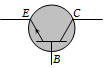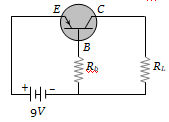For a transistor the parameter $\mathrm{\beta }$ = 99. The value of the parameter $\mathrm{\alpha }$ is

(a) 0.9                 (b) 0.99
(c) 1                    (d) 9

Concept Questions :-

Transistor
High Yielding Test Series + Question Bank - NEET 2020

Difficulty Level:

In a PNP transistor the base is the N-region. Its width relative to the P-region is

(a) Smaller           (b) Larger
(c) Same              (d) Not related

Concept Questions :-

Transistor
High Yielding Test Series + Question Bank - NEET 2020

Difficulty Level:

A common emitter amplifier is designed with NPN transistor ($\mathrm{\alpha }$ = 0.99). The input impedance is 1 K$\mathrm{\Omega }$ and load is 10 K$\mathrm{\Omega }$. The voltage gain will be

(a) 9.9                           (b) 99
(c) 990                          (d) 9900

Concept Questions :-

Applications of Transistor
High Yielding Test Series + Question Bank - NEET 2020

Difficulty Level:

The symbol given in figure represents(a) NPN transistor
(b) PNP transistor
(c) Forward biased PN junction diode
(d) Reverse biased NP junction diode

Concept Questions :-

Transistor
High Yielding Test Series + Question Bank - NEET 2020

Difficulty Level:

The most commonly used material for making transistor is

(a) Copper                 (b) Silicon
(c) Ebonite                 (d) Silver

Concept Questions :-

Transistor
High Yielding Test Series + Question Bank - NEET 2020

Difficulty Level:

An NPN-transistor circuit is arranged as shown in figure. It is(a) A common base amplifier circuit
(b) A common emitter amplifier circuit
(c) A common collector amplifier circuit
(d) Neither of the above

Concept Questions :-

Transistor
High Yielding Test Series + Question Bank - NEET 2020

Difficulty Level:

The part of a transistor which is heavily doped to produce a large number of majority carriers is-

1. Base

2. Emitter

3. Collector

4. None of these

Concept Questions :-

Transistor
High Yielding Test Series + Question Bank - NEET 2020

Difficulty Level:

For a transistor, the current amplification factor is 0.8. The transistor is connected in common emitter configuration. The change in the collector current when the base current changes by 6 mA is
(a) 6 mA                       (b) 4.8 mA
(c) 24 mA                     (d) 8 mA

Concept Questions :-

Transistor
High Yielding Test Series + Question Bank - NEET 2020

Difficulty Level:

In a common base amplifier circuit, calculate the change in base current if that in the emitter current is 2 mA and $\mathrm{\alpha }$ = 0.98

(a) 0.04 mA                           (b) 1.96 mA
(c) 0.98 mA                           (d) 2 mA

Concept Questions :-

Transistor
High Yielding Test Series + Question Bank - NEET 2020

Difficulty Level:

In a transistor circuit shown here the base current is 35 $\mu$A. The value of the resistor Rb is(a) 123.5 k$\mathrm{\Omega }$
(b) 257 k$\mathrm{\Omega }$
(c) 380.05 k$\mathrm{\Omega }$
(d) None of these

Concept Questions :-

Transistor